We think you are located in United States. Is this correct?

# Chapter 13: Types of reactions

In this chapter learners will explore acid-base reactions and redox reactions. Redox reactions were briefly introduced in gr10. The concepts of acids, bases, reduction, oxidation and oxidation numbers are all introduced here. The following list provides a summary of the topics covered in this chapter.

• Acids and bases.

This chapter begins by revising all the concepts done on acids and bases up to this point. Learners are reminded what an acid and a base are (in particular the Bronsted-Lowry definition) and how the definition and concept have changed over time. Although the most recent definition of an acid and a base is the Lowry definition this is not covered at school level and the Bronsted-Lowry definition serves as a good working model for the acids and bases that learners encounter at school.

The concept of a polyprotic acid is introduced although it is not in CAPS. This is done to help learners understand how to handle acids such as sulfuric acid in reactions. You should try to use polyprotic acids sparingly in your examples.

• Conjugate acids and bases and amphoteric (amphiprotic) substances.

The concept of conjugate acids and bases requires learners to think about reactions going in reverse. By writing the equation in reverse, learners can see how the acid becomes a base. This base is said to be the conjugate base of the acid since it is conjugated (linked) to the acid.

• Acid-hydroxide, acid-oxide and acid-carbonate reactions.

Three different types of bases are examined in detail to see how they react with acids. Several examples of each type are given and the general equation for the reaction is also given.

• Oxidation numbers for compounds.

This topic is placed after redox reactions in CAPS but must be taught before redox reactions and so is placed before redox reactions in this book. This topic provides the tools needed to understand redox reactions.

• Balancing redox reactions.

In grade 10 learners learnt how to balance chemical equations by inspection. In this topic they will learn how to balance redox reactions which often cannot be balanced by inspection. The simpler examples can be balanced by inspection and this can be used as a comparison for the two techniques. Learners need to be able to break a reaction up into two parts and follow different chemical species through an equation. This skill starts with conjugate acids and bases and carries over into this topic.

Coloured text has been used as a tool to highlight different parts of reactions. Ensure that learners understand that the coloured text does not mean there is anything special about that part of the reaction, this is simply a teaching tool to help them identify the important parts of the reaction.

It is also important to note that this chapter is split across term 3 and term 4. Acids and bases should be completed in term 3 and redox reactions are done in term 4.

All around you there are chemical reactions taking place. Green plants are photosynthesising, car engines are relying on the reaction between petrol and air and your body is performing many complex reactions. In this chapter we will look at two common types of reactions that can occur in the world around you and in the chemistry laboratory. These two types of reactions are acid-base reactions and redox reactions.

## Household acids and bases

Look around your home and school and find examples of acids and bases. Remember that foods can also be acidic or basic.

Make a list of all the items you find. Why do you think they are acids or bases?

Some common acids and bases, and their chemical formulae, are shown in Table 13.1.

 Acid Formula Base Formula Hydrochloric acid $$\text{HCl}$$ Sodium hydroxide $$\text{NaOH}$$ Sulfuric acid $$\text{H}_{2}\text{SO}_{4}$$ Potassium hydroxide $$\text{KOH}$$ Sulfurous acid $$\text{H}_{2}\text{SO}_{3}$$ Sodium carbonate $$\text{Na}_{2}\text{CO}_{3}$$ Acetic (ethanoic) acid $$\text{CH}_{3}\text{COOH}$$ Calcium hydroxide $$\text{Ca}(\text{OH})_{2}$$ Carbonic acid $$\text{H}_{2}\text{CO}_{3}$$ Magnesium hydroxide $$\text{Mg}(\text{OH})_{2}$$ Nitric acid $$\text{HNO}_{3}$$ Ammonia $$\text{NH}_{3}$$ Phosphoric acid $$\text{H}_{3}\text{PO}_{4}$$ Sodium bicarbonate $$\text{NaHCO}_{3}$$
Table 13.1: Some common acids and bases and their chemical formulae.

Most acids share certain characteristics, and most bases also share similar characteristics. It is important to be able to have a definition for acids and bases so that they can be correctly identified in reactions.

### Defining acids and bases (ESBQW)

One of the first things that was noted about acids is that they have a sour taste. Bases were noted to have a soapy feel and a bitter taste. However you cannot go around tasting and feeling unknown substances since they may be harmful. Also when chemists started to write down chemical reactions more practical definitions were needed.

A number of definitions for acids and bases have developed over the years. One of the earliest was the Arrhenius definition. Arrhenius (1887) noticed that water dissociates (splits up) into hydronium $$(\text{H}_{3}\text{O}^{+})$$ and hydroxide $$(\text{OH}^{-})$$ ions according to the following equation:

$$2\text{H}_{2}\text{O (l)} \rightarrow \text{H}_{3}\text{O}^{+}\text{(aq)} + \text{OH}^{-}\text{(aq)}$$

Arrhenius described an acid as a compound that increases the concentration of $$\text{H}_{3}\text{O}^{+}$$ ions in solution and a base as a compound that increases the concentration of $$\text{OH}^{-}$$ ions in solution.

Look at the following examples showing the dissociation of hydrochloric acid and sodium hydroxide (a base) respectively:

1. $$\text{HCl (aq)} + \text{H}_{2}\text{O}\text{(l)} \rightarrow \text{H}_{3}\text{O}^{+}\text{(aq)} + \text{Cl}^{-}\text{(aq)}$$

Hydrochloric acid in water increases the concentration of $$\text{H}_{3}\text{O}^{+}$$ ions and is therefore an acid.

2. $$\text{NaOH (s)} \stackrel{\text{H}_{2}\text{O}}{\longrightarrow} \text{Na}^{+}\text{(aq)} + \text{OH}^{-}\text{(aq)}$$

Sodium hydroxide in water increases the concentration of $$\text{OH}^{-}$$ ions and is therefore a base.

Note that we write $$\stackrel{\text{H}_{2}\text{O}}{\longrightarrow}$$ to indicate that water is needed for the dissociation.

However, this definition could only be used for acids and bases in water. Since there are many reactions which do not occur in water it was important to come up with a much broader definition for acids and bases.

In 1923, Lowry and Bronsted took the work of Arrhenius further to develop a broader definition for acids and bases. The Bronsted-Lowry model defines acids and bases in terms of their ability to donate or accept protons.

Acids

A Bronsted-Lowry acid is a substance that gives away protons (hydrogen cations $$\text{H}^{+}$$), and is therefore called a proton donor.

Bases

A Bronsted-Lowry base is a substance that takes up protons (hydrogen cations $$\text{H}^{+}$$), and is therefore called a proton acceptor.

Below are some examples:

1. $$\text{HCl (aq)} + \text{NH}_{3}\text{(aq)} \rightarrow \text{NH}_{4}^{+}\text{(aq)} + \text{Cl}^{-}\text{(aq)}$$

We highlight the chlorine and the nitrogen so that we can follow what happens to these two elements as they react. We do not highlight the hydrogen atoms as we are interested in how these change. This colour coding is simply to help you identify the parts of the reaction and does not represent any specific property of these elements.

$$\text{H}{\color{red}{\text{Cl}}} \text{ (aq)} + {\color{blue}{\text{N}}}\text{H}_{3}\text{(aq)} \rightarrow {\color{blue}{\text{N}}}\text{H}_{4}^{+}\text{(aq)} + {\color{red}{\text{Cl}}}^{-}\text{(aq)}$$

In order to decide which substance is a proton donor and which is a proton acceptor, we need to look at what happens to each reactant. The reaction can be broken down as follows:

$$\text{H}{\color{red}{\text{Cl}}}\text{ (aq)} \rightarrow {\color{red}{\text{Cl}}}^{-}\text{(aq)}$$ and

$${\color{blue}{\text{N}}}\text{H}_{3}\text{(aq)} \rightarrow {\color{blue}{\text{N}}}\text{H}_{4}^{+}\text{(aq)}$$

From these reactions, it is clear that $$\text{HCl}$$ is a proton donor and is therefore an acid, and that $$\text{NH}_{3}$$ is a proton acceptor and is therefore a base.

2. $$\text{CH}_{3}\text{COOH (aq)} + \text{H}_{2}\text{O (l)} \rightarrow \text{H}_{3}\text{O}^{+}\text{(aq)} + \text{CH}_{3}\text{COO}^{-}\text{(aq)}$$

Again we highlight the parts of the reactants that we want to follow in this reaction:

$${\color{red}{\text{CH}_{3}\text{COO}}}\text{H (aq)} + \text{H}_{2}{\color{blue}{\text{O}}}\text{ (l)} \rightarrow \text{H}_{3}{\color{blue}{\text{O}}}^{+}\text{(aq)} + {\color{red}{\text{CH}_{3}\text{COO}}}^{-}\text{(aq)}$$

The reaction can be broken down as follows:

$${\color{red}{\text{CH}_{3}\text{COO}}}\text{H (aq)} \rightarrow {\color{red}{\text{CH}_{3}\text{COO}}}^{-}\text{(aq)}$$ and

$$\text{H}_{2}{\color{blue}{\text{O}}}\text{ (l)} \rightarrow \text{H}_{3}{\color{blue}{\text{O}}}^{+}\text{(aq)}$$

In this reaction, $$\text{CH}_{3}\text{COOH}$$ (acetic acid or vinegar) is a proton donor and is therefore the acid. In this case, water acts as a base because it accepts a proton to form $$\text{H}_{3}\text{O}^{+}$$.

3. $$\text{NH}_{3}\text{(aq)} + \text{H}_{2}\text{O (l)} \rightarrow \text{NH}_{4}^{+}\text{(aq)} + \text{OH}^{-}\text{(aq)}$$

Again we highlight the parts of the reactants that we want to follow in this reaction:

$${\color{blue}{\text{N}}}\text{H}_{3}\text{(aq)} + \text{H}_{2}{\color{red}{\text{O}}}\text{ (l)} \rightarrow {\color{blue}{\text{N}}}\text{H}_{4}^{+}\text{(aq)} + {\color{red}{\text{O}}}\text{H}^{-}\text{(aq)}$$

The reaction can be broken down as follows:

$$\text{H}_{2}{\color{red}{\text{O}}}\text{ (l)} \rightarrow {\color{red}{\text{O}}}\text{H}^{-}\text{(aq)}$$ and

$${\color{blue}{\text{N}}}\text{H}_{3}\text{(aq)} \rightarrow {\color{blue}{\text{N}}}\text{H}_{4}^{+}\text{(aq)}$$

Water donates a proton and is therefore an acid in this reaction. Ammonia accepts the proton and is therefore the base.

Notice in these examples how we looked at the common elements to break the reaction into two parts. So in the first example we followed what happened to chlorine to see if it was part of the acid or the base. And we also followed nitrogen to see if it was part of the acid or the base. You should also notice how in the reaction for the acid there is one less hydrogen on the right hand side and in the reaction for the base there is an extra hydrogen on the right hand side.

#### Amphoteric substances

In examples $$\text{2}$$ and $$\text{3}$$ above we notice an interesting thing about water. In example $$\text{2}$$ we find that water acts as a base (it accepts a proton). In example $$\text{3}$$ however we see that water acts as an acid (it donates a proton)!

Depending on what water is reacting with it can either react as a base or as an acid. Water is said to be amphoteric. Water is not unique in this respect, several other substances are also amphoteric.

Amphoteric

An amphoteric substance is one that can react as either an acid or base.

When we look just at Bronsted-Lowry acids and bases we can also talk about amphiprotic substances which are a special type of amphoteric substances.

Amphiprotic

An amphiprotic substance is one that can react as either a proton donor (Bronsted-Lowry acid) or as a proton acceptor (Bronsted-Lowry base). Examples of amphiprotic substances include water, hydrogen carbonate ion ($$\text{HCO}_{3}^{-}$$) and hydrogen sulfate ion ($$\text{HSO}_{4}^{-}$$).

Note: You may also see the term ampholyte used to mean a substance that can act as both an acid and a base. This term is no longer in general use in chemistry.

temp text

#### Polyprotic acids [NOT IN CAPS]

A polyprotic (many protons) acid is an acid that has more than one proton that it can donate. For example sulfuric acid can donate one proton to form the hydrogen sulfate ion:

$\text{H}_{2}\text{SO}_{4}\text{(aq)} + \text{OH}^{-}\text{(aq)} \rightarrow \text{HSO}_{4}^{-}\text{(aq)} + \text{H}_{2}\text{O (l)}$

Or it can donate two protons to form the sulfate ion:

$\text{H}_{2}\text{SO}_{4}\text{(aq)} + 2\text{OH}^{-}\text{(aq)} \rightarrow \text{SO}_{4}^{2-}\text{(aq)} + 2\text{H}_{2}\text{O (l)}$

In this chapter we will mostly consider monoprotic acids (acids with only one proton to donate). If you do see a polyprotic acid in a reaction then write the resulting reaction equation with the acid donating all its protons.

Some examples of polyprotic acids are: $$\text{H}_{2}\text{SO}_{4}$$, $$\text{H}_{2}\text{SO}_{3}$$, $$\text{H}_{2}\text{CO}_{3}$$ and $$\text{H}_{3}\text{PO}_{4}$$.

## Acids and bases

Textbook Exercise 13.1

Identify the Bronsted-Lowry acid and the Bronsted-Lowry base in the following reactions:

$$\text{HNO}_{3}\text{(aq)} + \text{NH}_{3}\text{(aq)} \rightarrow \text{NO}_{3}^{-}\text{ (aq)} + \text{NH}_{4}^{+} \text{ (aq)}$$

We break the reaction into two parts:

$$\text{HNO}_{3}\text{ (aq)} \rightarrow \text{NO}_{3}^{-}\text{(aq)}$$ and

$$\text{NH}_{3}\text{(aq)} \rightarrow \text{NH}_{4}^{+}\text{(aq)}$$

From this we see that the Bronsted-Lowry acid is $$\text{HNO}_{3}$$ and the Bronsted-Lowry base is $$\text{NH}_{3}$$.

$$\text{HBr (aq)} + \text{KOH (aq)} \rightarrow \text{KBr (aq)} + \text{H}_{2}\text{O (l)}$$

We break the reaction into two parts:

$$\text{HBr (aq)} \rightarrow \text{KBr (aq)}$$ and

$$\text{KOH (aq)} \rightarrow \text{H}_{2}\text{O (l)}$$

From this we see that the Bronsted-Lowry acid is $$\text{HBr}$$ and the Bronsted-Lowry base is $$\text{KOH}$$.

Write a reaction equation to show $$\text{HCO}_{3}^{-}$$ acting as an acid.

$$\text{HCO}_{3}^{-}\text{ (aq)} \rightarrow \text{CO}_{3}^{2-}\text{(aq)} + \text{H}^{+}\text{(aq)}$$

Write a reaction equation to show $$\text{HCO}_{3}^{-}$$ acting as an base.

$$\text{HCO}_{3}^{-}\text{(aq)} + \text{H}^{+}\text{(aq)} \rightarrow \text{H}_{2}\text{CO}_{3}\text{(aq)}$$

Compounds such as $$\text{HCO}_{3}^{-}$$ are $$\ldots$$

Amphoteric

### Conjugate acid-base pairs (ESBQX)

Look at the reaction between hydrochloric acid and ammonia to form ammonium and chloride ions (again we have highlighted the different parts of the equation):

$${\color{red}{\text{HCl}}}\text{ (aq)} + {\color{blue}{\text{NH}_{3}}}\text{(aq)} \rightarrow {\color{blue}{\text{NH}_{4}^{+}}}\text{(aq)} + {\color{red}{\text{Cl}^{-}}}\text{(aq)}$$

We look at what happens to each of the reactants in the reaction:

$$\text{HCl (aq)} \rightarrow \text{Cl}^{-}\text{(aq)}$$ and

$$\text{NH}_{3}\text{(aq)} \rightarrow \text{NH}_{4}^{+}\text{(aq)}$$

We see that $$\text{HCl}$$ acts as the acid and $$\text{NH}_{3}$$ acts as the base.

But what if we actually had the following reaction:

$${\color{blue}{\text{NH}_{4}^{+}}}\text{(aq)} + {\color{red}{\text{Cl}^{-}}}\text{(aq)} \rightarrow {\color{red}{\text{HCl}}}\text{ (aq)} + {\color{blue}{\text{NH}_{3}}}\text{(aq)}$$

This is the same reaction as the first one, but the products are now the reactants.

Now if we look at the what happens to each of the reactants we see the following:

$$\text{NH}_{4}^{+}\text{(aq)} \rightarrow \text{NH}_{3}\text{(aq)}$$ and

$$\text{Cl}^{-}\text{(aq)} \rightarrow \text{HCl (aq)}$$

We see that $$\text{NH}_{4}^{+}$$ acts as the acid and $$\text{Cl}^{-}$$ acts as the base.

Up to now you have looked at reactions as starting with the reactants and going to the products. For acids and bases we also need to consider what happens if we swop the reactants and the products around. This will help you understand conjugate acid-base pairs.

When $$\text{HCl}$$ (the acid) loses a proton it forms $$\text{Cl}^{-}$$ (the base). And that when $$\text{Cl}^{-}$$ (the base) gains a proton it forms $$\text{HCl}$$ (the acid). We call these two species a conjugate acid-base pair. Similarly $$\text{NH}_{3}$$ and $$\text{NH}_{4}^{+}$$ form a conjugate acid-base pair.

The word conjugate means coupled or connected.

We can represent this as: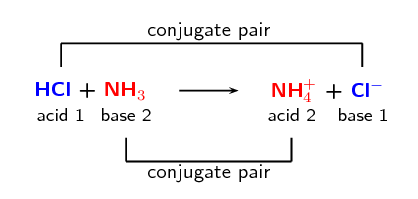## Conjugate acid-base pairs

Using the common acids and bases in Table 13.1, pick an acid and a base from the list. Write a chemical equation for the reaction of these two compounds.

Now identify the conjugate acid-base pairs in your chosen reaction. Compare your results to those of your classmates.

## Acids and bases

Textbook Exercise 13.2

In each of the following reactions, label the conjugate acid-base pairs.

$$\text{H}_{2}\text{SO}_{4}\text{(aq)} + \text{H}_{2}\text{O (l)} \rightarrow \text{H}_{3}\text{O}^{+}\text{(aq)} + \text{HSO}_{4}^{-}\text{(aq)}$$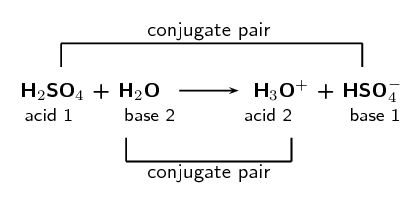$$\text{NH}_{4}^{+}\text{(aq)} + \text{F}^{-}\text{(aq)} \rightarrow \text{HF}\text{(aq)} + \text{NH}_{3}\text{(aq)}$$$$\text{H}_{2}\text{O (l)} + \text{CH}_{3}\text{COO}^{-}\text{(aq)} \rightarrow \text{CH}_{3}\text{COOH (aq)} + \text{OH}^{-}\text{(aq)}$$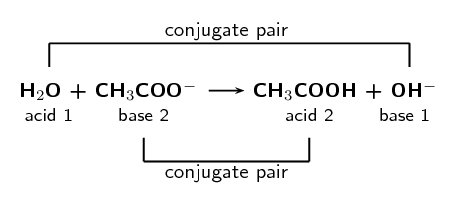$$\text{H}_{2}\text{SO}_{4}\text{(aq)} + \text{Cl}^{-}\text{(aq)} \rightarrow \text{HCl (aq)} + \text{HSO}_{4}^{-}\text{(aq)}$$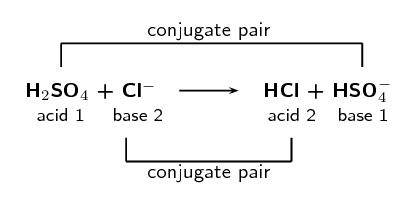Given the following reaction:

$\text{H}_{2}\text{O (l)} + \text{NH}_{3}\text{(aq)} \rightarrow \text{NH}_{4}^{+}\text{(aq)} + \text{OH}^{-}\text{(aq)}$

Write down which reactant is the base and which is the acid.

We break the reaction into two parts:

$$\text{H}_{2}\text{O (aq)} \rightarrow \text{OH}^{-}\text{(aq)}$$ and

$$\text{NH}_{3}\text{(aq)} \rightarrow \text{NH}_{4}^{+}\text{(aq)}$$

From this we see that the Bronsted-Lowry acid is $$\text{H}_{2}\text{O}$$ and the Bronsted-Lowry base is $$\text{NH}_{3}$$.

Label the conjugate acid-base pairs.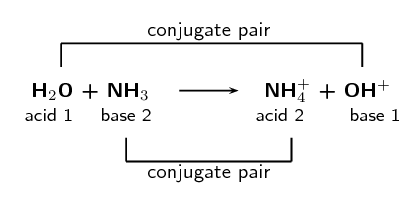In your own words explain what is meant by the term conjugate acid-base pair.

A conjugate acid-base pair is a reactant and product pair that is transformed into each other through the loss or gain of a proton. So for example an acid loses a proton to form a base. The acid and the resulting base are said to be a conjugate acid-base pair.